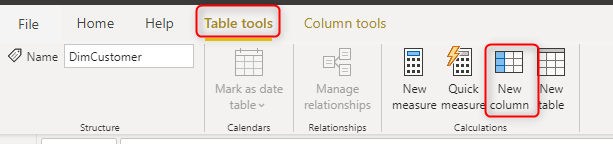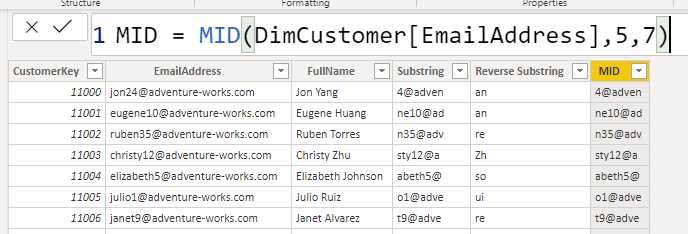# Substring in DAX: How to get Part of String Field in Power BI Using DAX ExpressionSubstring is one of the most common functions in many languages, However, there is no function named Substring DAX. There is a very simple way of doing it, which I am going to explain in this post. Substring means getting part of a string, for example from “Reza Rad”, if I want to get the start starting from index 2, for 4 characters, it should return “za R”. Considering that the first character is index 0. Let’s see how this is possible.

## Sample Data

I have a sample customer table as below;## Substring

Substring means saying from character indexed N, extract M characters: Substring (N,M)

This can be implemented in DAX in different ways, this is one of the methods:

```Substring = LEFT(
RIGHT(
),
3)```

This added as a column to this table,will produce characters starting from index 1 for the length of 3.1, in the expression above, is the starting index. if you want to start from the beginning of the text, use zero here.

3, in the expression above, is the length of the output from the starting index.

here is another example:There is an easier way to do substring too, using MID function;

`MID = MID(DimCustomer[EmailAddress],5,7)`Using the MID function, you just specify the starting index, and the length of characters to extract, similar to substring in many other languages.

## Reverse Substring

Sometimes you want substring to start from the end of the text. For example, ReverseSubString (N, M) means to start from N which is the index from the right end of the string and extract M characters. For example, for “Reza Rad”, with ReverseSubstring(3,2), means “Ra”. You can implement the Reverse substring as below:

```Reverse Substring = LEFT(
RIGHT(DimCustomer[FullName],3)
,2)```

the result is:This method is usually more useful when the value you want to extract is closer to the end of the string rather than the start.

I hope this little script can help in your DAX expressions, if you have any questions, feel free to write a comment below.